This is “Accounting for Zero-Coupon Bonds”, section 14.3 from the book Business Accounting (v. 2.0). For details on it (including licensing), click here.

Has this book helped you? Consider passing it on:
Creative Commons supports free culture from music to education. Their licenses helped make this book available to you.
DonorsChoose.org helps people like you help teachers fund their classroom projects, from art supplies to books to calculators.

## 14.3 Accounting for Zero-Coupon Bonds

### Learning Objectives

At the end of this section, students should be able to meet the following objectives:

1. Identify the characteristics of a zero-coupon bond.
2. Explain how interest is earned by a creditor on a zero-coupon bond.
3. Understand the method of arriving at an effective interest rate for a bond.
4. Calculate the price of a zero-coupon bond and list the variables that affect this computation.
5. Prepare journal entries for a zero-coupon bond using the effective rate method.
6. Explain the term “compounding.”
7. Describe the theoretical problems associated with the straight-line method, and identify the situation in which this method can be applied.

## The Issuance of a Zero-Coupon Bond

Question: A wide array of bonds and other types of financial instruments can be issued by parties (businesses and other organizations) seeking money. A zero-coupon bond is one that is popular because of its ease. The face value of a zero-coupon bond is paid to the creditor after a specified period of time, but no further cash is ever exchanged. Thus, no stated cash interest rate is paid. Money is received when the bond is issued and money is paid at the end of the term but no other payments are made. Why does any investor choose to purchase a zero-coupon bond if no interest is paid?

Answer: No investor would buy a note or bond that did not pay interest. That makes no economic sense. Because zero-coupon bonds are widely issued, some form of interest must be included.

These bonds are sold at a discount below face value with that reduction serving as interest. If a bond is issued for \$37,000 and the company eventually repays its face value of \$40,000, the \$3,000 difference is the interest on the debt. That additional payment is the charge paid for the use of the money that was borrowed. The price reduction below face value can be so significant that zero-coupon bonds are sometimes referred to as deep discount bonds.

To illustrate, assume that on January 1, Year One, a company offers a \$20,000 two-year zero-coupon bondA debt instrument that includes no interest payments; these bonds are issued at a discount with the difference between the cash received at the beginning and the cash paid on the maturity date serving as interest for that period of time. to the public. A single payment of \$20,000 will be made to the holder of this bond on December 31, Year Two. According to the contract, no other cash will be paid. An investor who wishes to earn 7 percent annual interest can mathematically compute the exact amount to bid for this contract. The debtor must then decide whether to accept this offer.

Often, the actual exchange price for a bond is the result of a serious negotiation process to establish the interest rate to be earned. The potential creditor might initially offer an amount that equates to interest at an annual rate of 7 percent. The debtor could then counter by suggesting that an annual rate of 5 percent is more reasonable. After some discussion, the two parties might compromise by settling on a price that provides an annual interest rate of 6 percent. In the bond market, interest rates are the subject of intense negotiations. After the effective rateThe interest rate determined by negotiation and market forces that is used to set the price of a bond; it is also called the yield rate and often varies from the interest rate stated on the indenture that is used to establish cash interest payments. (also called the yield or negotiated rate) has been agreed on by both parties, the actual price of the bond is simply a mathematical computation.

## Agreeing on a Price for a Bond

Question: A \$20,000 zero-coupon bond is being issued by a company. According to the indenture, it comes due in exactly two years. The parties have negotiated an annual interest rate to be earned of 6 percent. How is the actual price to be paid for a bond mathematically determined after an effective rate of interest has been established?

Answer: Calculation of the price of a bond is based on the present value of the cash payments in the same manner as demonstrated previously in the coverage of intangible assets. Future cash payments are first identified and then valued by the mathematical removal of interest (the present value computation).

Here, a single cash payment of \$20,000 is to be made by the debtor in two years. The parties have negotiated an annual 6 percent effective interest rate. Thus, a portion of the future cash (\$20,000) serves as interest at an annual rate of 6 percent for this two-year period of time. In a present value computation, interest at the designated rate is calculated and subtracted to leave the principal amount of those payments. That is the price of the bond. Interest at a 6 percent annual rate for two years is removed. The remainder is the amount paid for the bond.

### Present Value of \$1

http://www.principlesofaccounting.com/ART/fv.pv.tables/pvof1.htm

The present value of \$1 in two years at an annual rate of interest of 6 percent is \$0.8900. This can be found in a chart (through the link presented or in the tables at the end of this book), by formula, or by use of an Excel spreadsheet.As explained in the chapter on acquiring intangible assets, the present value of \$1 can be mathematically determined using the formula \$1/(1 + i)n. Here, i is 0.06 and n is two periods. Present value can also be determined using an Excel spreadsheet. If a spreadsheet is used, the present value of \$1 at 6 percent in two periods is found by typing the following formula into a cell: =PV(.06,2,,1,0). Because the actual payment is \$20,000 and not \$1, the present value of the cash flows from this bond (its price) can be found as follows:

present value = future cash payment × \$0.8900 present value = \$20,000 × \$0.8900 present value = \$17,800

Bond prices are often stated as a percentage of face value. Thus, this bond is sold to the investor at “89” (\$17,800/\$20,000), which indicates that the price is 89 percent of the \$20,000 face value. The price is the future cash payments with the negotiated rate of interest removed. If the investor pays \$17,800 today and the debtor returns \$20,000 in two years, the additional \$2,200 is the interest. Mathematically, this extra \$2,200 is equal to interest at 6 percent per year.

The issuance is recorded through the entry presented in Figure 14.8 "January 1, Year One—Zero-Coupon Bond Issued at Effective Annual Interest Rate of 6 Percent".The entry shown in Figure 14.8 "January 1, Year One—Zero-Coupon Bond Issued at Effective Annual Interest Rate of 6 Percent" can also be recorded in a slightly different manner. Under this alternative, the liability is entered into the records at its face value of \$20,000 along with a separate discount of \$2,200. The discount serves as a contra account to reduce the net liability balance to its principal amount. Although visually different, the liability is still reported as \$17,800.

Figure 14.6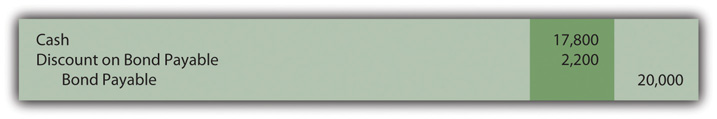Figure 14.8 January 1, Year One—Zero-Coupon Bond Issued at Effective Annual Interest Rate of 6 Percent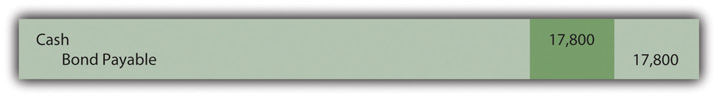### Test Yourself

Question:

On January 1, Year One, the Leisinger Company offers a ten-year zero-coupon bond with a face value of \$80,000 to an investor. Leisinger hopes to issue this bond at an annual interest rate of 4 percent. The investor wants 7 percent and they finally agree on 5 percent. The present value of \$1 at 4 percent annual interest for 10 years is \$0.6756 and at 5 percent for ten years is \$0.6139. The present value of an ordinary annuity of \$1 for ten years at 4 percent annual interest is \$8.1109 and at 5 percent is \$7.7217. What amount does Leisinger Company receive (rounded)?

1. \$49,112
2. \$54,048
3. \$61,774
4. \$64,887

The correct answer is choice a: \$49,112.

Explanation:

The negotiated effective (or yield) interest rate is 5 percent per year. A single payment will be made in ten years. Using the rate for \$1 (a single amount) in ten years at 5 percent interest per year, the present value is the cash of \$80,000 times \$0.6139 or \$49,112. The present value factors have been mathematically determined to compute the appropriate interest which is then removed to leave the principal. At an annual rate of 5 percent, \$49,112 will grow to be exactly \$80,000 in ten years.

## Recognizing Interest on a Zero-Coupon Bond

Question: The \$20,000 zero-coupon bond discussed above is issued for \$17,800 so that a 6 percent annual interest rate will be earned. As shown in Figure 14.8 "January 1, Year One—Zero-Coupon Bond Issued at Effective Annual Interest Rate of 6 Percent", the bond is initially recorded at this principal amount.

Subsequently, two problems must be addressed by the accountant.

• First, the company actually has to pay \$20,000. Over time, the \$17,800 principal balance must be raised to that face value. At the end of Year Two, the liability should be reported as \$20,000.
• Second, the \$2,200 difference between the amount received and the eventual repayment (\$20,000 less \$17,800) has to be recognized as interest expense over these two years. The additional payment is the cost of the debt for this period, the interest.

To arrive at fairly presented figures, both of these problems must be resolved. How is a zero-coupon bond reported in the period after its issuance?

Answer: In the earlier discussion of present value and the purchase of assets (such as a patent), the effective rate method was demonstrated. It solves the two accounting problems mentioned here. The reported debt balance is raised gradually to the face value and interest expense equal to 6 percent is reported each year until maturity.

Interest recognized for Year One should be the \$17,800 principal balance multiplied by the effective interest rate of 6 percent or \$1,068. However, no cash interest payment is made for this zero-coupon bond. Thus, as is shown in Figure 14.9 "December 31, Year One—Interest on Zero-Coupon Bond at 6 Percent Rate Is Recognized and Compounded", this interest is compounded—added to the principal of the debt. Interest that is recognized but not paid at that time is compounded, included in the balance of the liability.

Figure 14.9 December 31, Year One—Interest on Zero-Coupon Bond at 6 Percent Rate Is Recognized and CompoundedIf a discount is recorded in the initial entry, as is shown in endnote 6, the credit shown in Figure 14.9 "December 31, Year One—Interest on Zero-Coupon Bond at 6 Percent Rate Is Recognized and Compounded" is to the Discount account and not directly to the bond payable. Because the contra account is reduced, the net liability balance increases by \$1,068. Thus, overall reporting of the interest and the liability is not impacted by the method used in recording the issuance of the bond.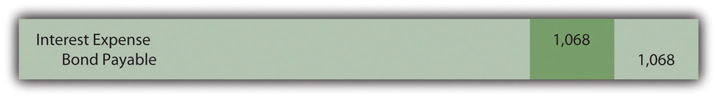The compounding of this interest raises the principal by \$1,068 from \$17,800 to \$18,868. The balances to be reported in the financial statements at the end of Year One are as follows:

 Year One—Interest Expense (Income Statement) \$1,068 December 31, Year One—Bond Payable (Balance Sheet) \$18,868

The principal is higher in Year Two because of the compounding (addition) of the first year interest. As the principal increases, interest for subsequent periods must also go up. As reflected in Figure 14.10 "December 31, Year Two—Interest on Zero-Coupon Bond at 6 Percent Rate Is Recognized and Compounded", interest for Year Two is 6 percent of the new liability balance of \$18,868 or \$1,132 (rounded).

Figure 14.10 December 31, Year Two—Interest on Zero-Coupon Bond at 6 Percent Rate Is Recognized and Compounded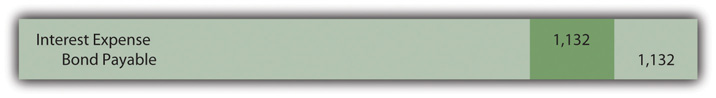Note that the bond payable balance has now been raised to \$20,000 as of the date of payment (\$17,800 + \$1,068 + \$1,132). In addition, interest expense of \$2,200 (\$1,068 + \$1,132) has been recognized over the two years. Reported interest was exactly 6 percent of the principal in each year. Total interest reported for this zero-coupon bond is equal to the difference between the amount received by the debtor and the face value eventually repaid. Both of the accounting problems have been resolved through the application of the effective rate method.

The \$17,800 price of the bond was computed mathematically based on the following:

• The cash payment (\$20,000)
• The time periods (two)
• The effective rate of interest (the 6 percent negotiated rate)
• The pattern of cash flows (a single payment in the future)

Interest is then recognized each period based on this same set of variables. Thus, the resulting numbers will reconcile. Interest expense reported for the two years is \$2,200 and the final liability balance comes back to \$20,000.

### Test Yourself

Question:

The O’Neil Company issues a six-year \$40,000 zero-coupon bond on January 1, Year One. At that time, most companies are able to borrow money at an annual rate of 10 percent. O’Neil is in such good financial health that the bond is sold for \$25,207 to yield a negotiated rate of 8 percent per year. What is reported in O’Neil’s financial statements at the end of Year One if the effective rate method is applied? (All numbers are rounded.)

1. Interest expense of \$2,017 and a bond payable of \$27,224.
2. Interest expense of \$2,112 and a bond payable of \$27,319.
3. Interest expense of \$2,215 and a bond payable of \$27,422.
4. Interest expense of \$2,521 and a bond payable of \$27,728.

The correct answer is choice a: Interest expense of \$2,017 and a bond payable of \$27,224.

Explanation:

Interest to be reported for the first year is the 8 percent negotiated rate times the principal of \$25,207 or \$2,017 (rounded). This is a zero-coupon bond so no interest is paid as time passes. Because no cash interest is actually paid, this entire amount of interest for the first year is compounded to raise the principal by \$2,017 from \$25,207 to \$27,224. In this manner, the principal balance should reach \$40,000 after six years.

### Test Yourself

Question:

Johnson Company issues an eight-year \$50,000 zero-coupon bond on January 1, Year One. After serious negotiations between the company and the investor, this bond is sold for \$31,371 to yield an effective annual rate of 6 percent. What is reported in Johnson’s financial statements at the end of Year Two if the effective rate method is applied? (All numbers are rounded.)

1. Interest expense of \$1,882 and a bond payable of \$33,253.
2. Interest expense of \$1,882 and a bond payable of \$35,135.
3. Interest expense of \$1,995 and a bond payable of \$35,248.
4. Interest expense of \$2,015 and a bond payable of \$35,285.

The correct answer is choice c: Interest expense of \$1,995 and a bond payable of \$35,248.

Explanation:

Interest for the first year is the \$31,371 principal times the effective annual rate of 6 percent or \$1,882. As a zero-coupon bond, no interest is paid. The entire \$1,882 is compounded by adding it to the principal so that the balance is \$33,253. For Year Two, interest expense is now recognized as \$1,995 or 6 percent times the updated principal of \$33,253. Again, the interest is compounded so the liability is reported at \$35,248. In this manner, the balance will be \$50,000 after 8 years.

## Recognizing Interest Using the Straight-Line Method

Question: The previous zero-coupon bond was sold at the present value of its future cash flows based on a rate of interest negotiated by the parties involved. Interest was then recognized each year by applying the effective rate method. Is the effective rate method the only acceptable technique that can be used to compute and report interest when the face value of a debt differs from its issue price?

Answer: When a zero-coupon bond is issued at a discount, interest to be reported each year can also be calculated by a simpler approach known as the straight-line method. According to this technique, an equal amount of the discount is assigned to interest each period over the life of the bond. This zero-coupon bond was issued for \$2,200 below face value to provide interest to the buyer. Payment of the face value will be made in two years. As is shown in Figure 14.11 "December 31, Years One and Two—Interest on Zero-Coupon Bond at 6 Percent Rate—Straight-Line Method", the straight-line method recognizes interest of \$1,100 annually (\$2,200/2 years).

Figure 14.11 December 31, Years One and Two—Interest on Zero-Coupon Bond at 6 Percent Rate—Straight-Line Method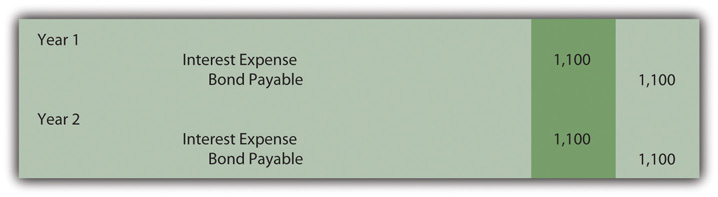Once again, the bond payable balance has been raised to \$20,000 by the end of the second year (\$17,800 + \$1,100 + \$1,100) and total interest expense over the life of the bond equals the \$2,200 discount (\$1,100 + \$1,100). However, a theoretical question should be raised as to whether the information reported under this method is a fairly presented portrait of the events that took place. Although the bond was sold to earn 6 percent annual interest, this rate is not reported for either period.

Year One: \$1,100 interest/\$17,800 principal = 6.2 percent

Compounding of the interest raises the principal by \$1,100 to \$18,900

Year Two: \$1,100 interest/\$18,900 principal = 5.8 percent

The debtor and creditor agreed on an annual rate of exactly 6 percent for the entire two-year period. When applying the straight-line method, this rate is not reported for either year. Furthermore, the interest rate appears to float (6.2 percent to 5.8 percent) as if a different rate was negotiated for each year. That change does not reflect reality. A single 6 percent annual interest rate was established by these two parties.

Although the straight-line method creates some theoretical concerns, it can still be applied according to U.S. GAAP but only if the reported results are not materially different from those derived using the effective rate method.

### Test Yourself

Question:

The Reynolda Company issues an eight-year \$80,000 zero-coupon bond on January 1, Year One. After serious negotiations, this bond is sold for \$54,144 to yield an effective annual rate of 5 percent. What is reported in Reynolda’s financial statements at the end of Year Two if the straight-line method is applied to this discount? (All numbers are rounded.)

1. Interest expense of \$3,198 and a bond payable of \$60,540.
2. Interest expense of \$3,232 and a bond payable of \$60,608.
3. Interest expense of \$3,268 and a bond payable of \$60,680.
4. Interest expense of \$3,304 and a bond payable of \$60,752.